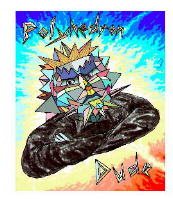Acronym sne Name snub icosiicosahedron,compound of 5 ike `© ©` Circumradius sqrt[(5+sqrt(5))/8] = 0.951057 Inradius sqrt[(7+3 sqrt(5))/24] = 0.755761 Vertex figure  Dihedral angles (at margins) between {3} and {3}:   arccos(-sqrt(5)/3) = 138.189685° ExternallinksFaces of an octahedral subset of triangles each, pairwise fall into coincident face planes. So either those can be considered separately (type A); or they are considered as (rotated) 2-triangle-compounds (type B).

Incidence matrix

```(Type A)

60 |   4  1 |  3  2 || 1
-----+--------+-------++--
2 | 120  * |  1  1 || 1
2 |   * 30 |  2  0 || 1
-----+--------+-------++--
3 |   2  1 | 60  * || 1
3 |   3  0 |  * 40 || 1
-----+--------+-------++--
♦ 12 |  24  6 | 12  8 || 5
```

```(Type B)

60 |   4  1 |  3  2 || 1
-----+--------+-------++--
2 | 120  * |  1  1 || 1
2 |   * 30 |  2  0 || 1
-----+--------+-------++--
3 |   2  1 | 60  * || 1
6 |   6  0 |  * 20 || 2
-----+--------+-------++--
♦ 12 |  24  6 | 12  8 || 5
```